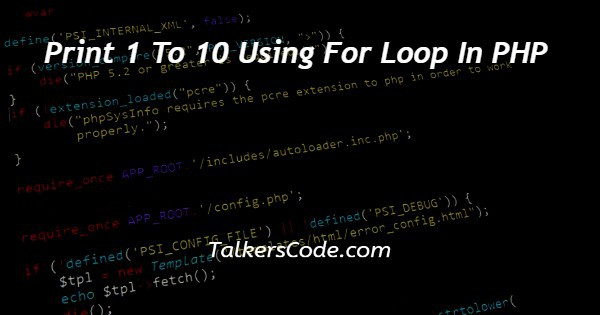# Print 1 To 10 Using For Loop In PHP

Last Updated : Jan 1, 2023In this tutorial we will show you the solution of print 1 to 10 using for loop in PHP, when printing more than two values as we know we have to use loop functions, in php for(), foreach() and while(), etc.., are available.

When we have to code same process till some condition we can move with loops otherwise it takes too much time, space of code and had complexities.

Here we need to use for loop for printing numbers from one to ten. In loop always have number of executions available till condition fails, increment steps and some body of codes.

## Step By Step Guide On Print 1 To 10 Using For Loop In PHP :-

Here we used for loop that means a loop variable is used to control the loop. First initialize this loop variable to some value, then check whether this variable is less than or greater than counter value.

If statement is true, then loop body is executed and loop variable gets updated. Steps are repeated till exit condition comes. Same as other loop also but the syntax only vary.

```<?php
for(\$i=1;\$i<=10;\$i++){
echo \$i.'<br>';
}
?>```
1. A php script can be placed anywhere in the document. A php script starts with <?php and end with ?>.
2. The default file extension for php files is “.php” and php statements end with ‘;’ semicolon.
3. First we need to create for loop with one variable here declared variable ‘\$i’ with value ‘1’ and ‘\$’ symbol must add before variable name when we define a variable in php.
4. For loop syntax is, for(variable_name=start value;variable_name condition end value;variable increament){ our code }
5. Now we can get some idea about our program, variable \$i to value ‘1’ defines we need to print from number ‘1’ so we initialized from ‘1’.
6. This loop needs to work till number ‘10’ so we gives condition as upto ‘\$i<=10’ one thing is near less than symbol equal to symbol is must because we need to print number ‘10’ also. If we gives only less than means then output will print up to ‘9’.
7. Because in loop its checks after variable \$i updated as value ‘10’ condition (10<10) fails so it can print value ‘10’.
8. The ‘\$i++’ is increment for each time it is increased by ‘1’ till loop ends. Our program first prints ‘1’ to webpage then increased and checks condition then printed likewise this loop done.
9. Results printed on webpage browser that <br> for after each time value printed it points next line so output will looks clear otherwise we can’t see clearly.

## Conclusion :-

In conclusion we are able to know how to covert stdclass object to array using php.

When work with php we need to create and process php files at server location and then we need to start the server before execute the program.

When we executing this program on browser it prints first value of initialized value when it first execution in for loop it initialized and condition checks then body of code will work after that execution only do another step of increment then condition checks and body code will work it same as working till condition fails.

Here our body code is echo() so each time variable ‘\$i’ value printed with break on webpage.

I hope this tutorial on print 1 to 10 using for loop in PHP helps you and the steps and method mentioned above are easy to follow and implement.